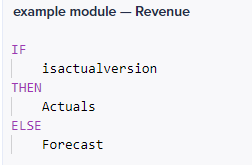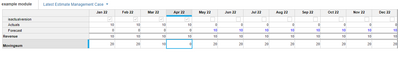# Movingsum over if statement based on isactualversion()Hi all!

In our model we do not get the wished results from a moving sum.

I want to preform a movingsum over a line item which is constructed with an if statement in a model with versions.

This is my line item if statement:Where isactualversion is iscactualversion() and is thus dependent on the version.

Then we have a line with a movingsum over the line item showed above:This gives not the answer I expect for the last two actual months.

In the case we set a value of 10 for each each month (Actuals and Forecast) I expect the result of the sum to be 20 for each month. But the results are as follows:The last two actuals months (Mar 22 and Apr 22) give the results as if summed over the actuals only instead over the combination of actual and forecast.

How can I construct the moving sum such that it actually sums the values I see in the Revenue line item?

Tagged: